# Geometrical Progression

A series of numbers is in geometrical progression if each number in the series is a constant multiple of its preceding number. As an example, Macdonald gives the series 2, 8, 32, 128, 512, 2048, 8192, etc. so that the constant multiple is 4. The notes jump immediately into finding the sum of series in geometrical progression. When the general form of the sum is given by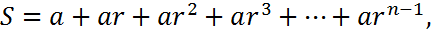where is the first term in the series, r is the ratio between succeeding terms, n is the number of terms in the sum and S is value of the sum. Macdonald derives the value for S in the usual way. Multiply both sides of the equation by r, find the difference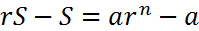, and solve for S to get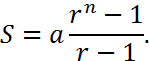In the initial numerical example, a = 2, r = 4, and n is not given. Up to the “etc.” in the series, n = 7 in which case S = 10922.

In arithmetic textbooks and cyphering books of this era, the usual application of geometrical progressions is to the valuation of annuities at compound interest. If interest is paid at a rate of per annum, then capital of 1 invested at the rate i per annum will accumulate to 1 + i at the end of one year and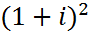at the end of two years (interest is earned on interest). By the same concept a value of 1 payable at the end of one year is worth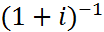now and a value of payable at the end of two years is worth now. This can be generalized to the value of an annuity that pays at the end of every year for years. It is worth in today’s money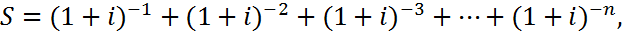which is a geometrical progression with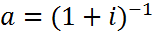and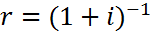. Macdonald does not treat interest and annuities. In fact, no form of commercial arithmetic appears in the notebook. The whole notebook is an academic exercise rather than training to become adept at commercial arithmetic.

The three examples that Macdonald does treat are given as word problems. They all originate in Nicholas Pike’s A New and Complete System of Arithmetick. George Baxter picked, and slightly edited, the more interestingly worded examples among the ones that were available. The first two problems are to find the sum of the series; the third problem is to find the last term in the series.

1. A gentleman, whose daughter was married on New Year’s day, gave her a guinea, promising to triple his gift on the first day of the month in the year. What is the ladies [sic] fortune?